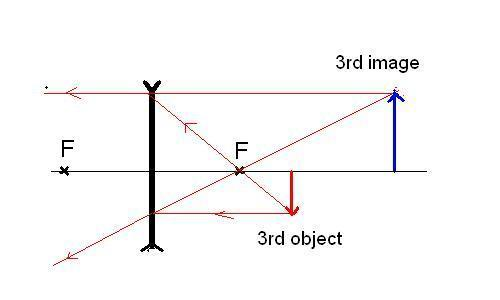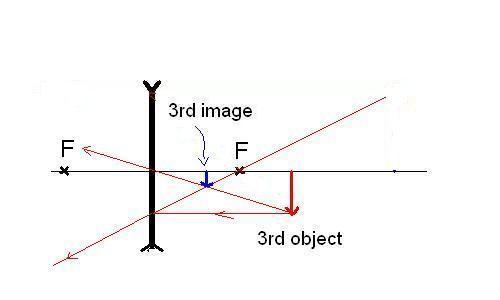# Optical System with Diverging Lens and Concave Mirror

## Homework Statement

A diverging thin lens and a concave mirror have focal lengths of equal magnitude. An object is placed (3/2)f from the diverging lens and the mirror is placed a distance 3f on the other side of the lens. Using Gaussian optics, determine the final image of the system after two refractions. (Pedrotti-3, 2-22)

## Homework Equations

$$\frac{1}{s}+\frac{1}{s'}=\frac{1}{f}$$

## The Attempt at a Solution

I'm having trouble getting the final answer. After the first refraction, s' = -3f/5 so it is to the left of the lens. Then after the reflection, s' = 18f/13 so it is to the left of the mirror and to the right of the lens. So now in the last refraction, s = 3f - 18f/13 = 21f/13 but it should be negative since it is to the right of the lens. After using the formula (s = -21f/13, f = -f), I get s' =-21/8 so it is to the right of the lens but the answer should be: Final image between lens and mirror at 21f/34 from lens. If s = +21f/13 then it will work out. Why is it positive instead of negative even though the object is to the right of the lens before the second refraction?

ehild
Homework Helper
The second image is real, and it is to the right of the lens. The sign of the object distance is determined with respect to the incoming light. It is a real object for the other side of the lens. The image is virtual, but for that situation, virtual means to the right of the lens.

Edit: Wrong ray diagram.Correct one:Last edited:
Simon Bridge
Homework Helper
I think there's a double negative in there because the object is to the right and the lens is diverging or something.
Go through it carefully. Image distances to the right of the lens are positive right? A check-list often helps.

Personally I do this by sketching the ray diagram - it fits on a page.
I can never remember the sign conventions.

 ehild beat me to it ... moral: check with ray diagrams.

Also, there are rules for drawing the ray diagrams when the light rays come from the left to the right. How are you drawing the ray diagram when the light rays are coming from the right?

ehild
Homework Helper
Imagine you keep a magnifying glass in your hand. You can illuminate it with a torch either from left or from right can you not? And then you get a real image on the other side - on the right side in the first case and on the left side in the second case. Why do you think that the light must come from the left always???????
The same rules apply in both cases. Use "front" and "behind" instead of left and right.

If it is a converging lens, the rays parallel with the axis of the lens go through the focus behind the lens.
In case of a diverging lens, the parallel rays travel behind the lens as if they emerged from the front-side focus.

Last edited:
Imagine you keep a magnifying glass in your hand. You can illuminate it with a torch either from left or from right can you not? And then you get a real image on the other side - on the right side in the first case and on the left side in the second case. Why do you think that the light must come from the left always???????

It's just because the formula 1/s + 1/s' = 1/f used with the standard sign convention requires that the light rays must come from the left. Also I think your ray diagram is wrong (if you were drawing it to represent the the final image in the original question)

ehild
Homework Helper
There are quite a few standard sign conventions for lenses :) Try to think of a real lens and a real situation.
Yes, my ray diagram is wrong. Sorry. Can you tell please, how to correct it? :)